## k-means聚类算法k-means是Lloyd博士在1957年提出的，虽然这个算法已有50年的历史，但却是当前最流行的聚类算法！1. 我们想将图中的记录分成三个分类（即k=3），比如上文提到的犬种数据，坐标轴分别是身高和体重。
2. 由于k=3，我们随机选取三个点来作为聚类的起始点（分类的中心点），并用红黄蓝三种颜色标识。
3. 然后，我们根据其它点到中心点的距离来进行分配，这样就能将这些点分成三类了。
4. 计算这些分类的中心点，以此作为下一次计算的起始点。重复这个过程，直到中心点不再变动，或迭代次数超过某个阈值为止。

1. 随机选取k个元素作为中心点；
2. 根据距离将各个点分配给中心点；
3. 计算新的中心点；
4. 重复2、3，直至满足条件。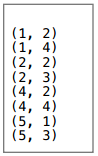(1 + 1 + 2) / 3 = 4 / 3 = 1.33

y轴是：

(2 + 4 + 3) / 3 = 9 / 3 = 3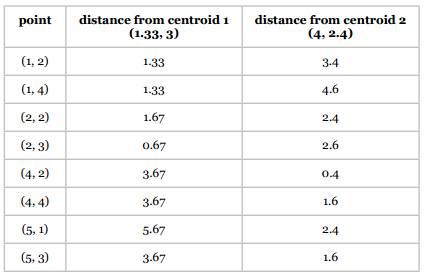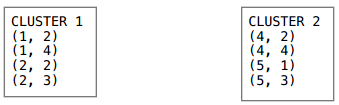• 分类1：(1.5, 2.75)
• 分类2：(4.5, 2.5)• 分类1：(1.5, 2.75)
• 分类2：(4.5, 2.5)k-means好简单呀！

k-means是一种最大期望算法，这类算法会在“期望”和“最大化”两个阶段不断迭代。比如k-means的期望阶段是将各个点分配到它们所“期望”的分类中，然后在最大化阶段重新计算中心点的位置。如果你对此感兴趣，可以前去阅读维基百科上的词条。

### 登山式算法1. 在山上随机选取一个点作为开始；
2. 向高处爬一点；
3. 重复第2步，直到没有更高的点。k-means就是这样一种算法，它不能保证最终结果是最优的，因为我们一开始选择的中心点是随机的，很有可能就会选到上面的A点，最终获得局部最优解B点。因此，最终的聚类结果和起始点的选择有很大关系。但尽管如此，k-means通常还是能够获得良好的结果的。### 误差平方和（SSE）``````import math
import random

"""
K-means算法
"""

def getMedian(alist):
"""计算中位数"""
tmp = list(alist)
tmp.sort()
alen = len(tmp)
if (alen % 2) == 1:
return tmp[alen // 2]
else:
return (tmp[alen // 2] + tmp[(alen // 2) - 1]) / 2

def normalizeColumn(column):
"""计算修正的标准分"""
median = getMedian(column)
asd = sum([abs(x - median) for x in column]) / len(column)
result = [(x - median) / asd for x in column]
return result

class kClusterer:
"""kMeans聚类算法，第一列是分类，其余列是数值型特征"""

def __init__(self, filename, k):
"""k是分类的数量，该函数完成以下功能：
1. 读取filename的文件内容
2. 按列存储到self.data变量中
3. 计算修正的标准分
4. 随机选取起始点
5. 将各个点分配给中心点
"""
file = open(filename)
self.data = {}
self.k = k
self.counter = 0
self.iterationNumber = 0
# 用于跟踪本次迭代有多少点的分类发生了变动
self.pointsChanged = 0
# 误差平方和
self.sse = 0
#
# 读取文件
#
file.close()
self.data = [[] for i in range(len(header))]
# 按列存储数据，如self.data是第一列的数据，
# self.data是第一列第十行的数据。
for line in lines[1:]:
cells = line.split(',')
toggle = 0
for cell in range(self.cols):
if toggle == 0:
self.data[cell].append(cells[cell])
toggle = 1
else:
self.data[cell].append(float(cells[cell]))

self.datasize = len(self.data)
self.memberOf = [-1 for x in range(len(self.data))]
#
# 标准化
#
for i in range(1, self.cols):
self.data[i] = normalizeColumn(self.data[i])

# 随机选取起始点
random.seed()
self.centroids = [[self.data[i][r]  for i in range(1, len(self.data))]
for r in random.sample(range(len(self.data)),
self.k)]
self.assignPointsToCluster()

def updateCentroids(self):
"""根据分配结果重新确定聚类中心点"""
members = [self.memberOf.count(i) for i in range(len(self.centroids))]
self.centroids = [[sum([self.data[k][i]
for i in range(len(self.data))
if self.memberOf[i] == centroid])/members[centroid]
for k in range(1, len(self.data))]
for centroid in range(len(self.centroids))]

def assignPointToCluster(self, i):
"""根据距离计算所属中心点"""
min = 999999
clusterNum = -1
for centroid in range(self.k):
dist = self.euclideanDistance(i, centroid)
if dist < min:
min = dist
clusterNum = centroid
# 跟踪变动的点
if clusterNum != self.memberOf[i]:
self.pointsChanged += 1
# 计算距离平方和
self.sse += min**2
return clusterNum

def assignPointsToCluster(self):
"""分配所有的点"""
self.pointsChanged = 0
self.sse = 0
self.memberOf = [self.assignPointToCluster(i)
for i in range(len(self.data))]

def euclideanDistance(self, i, j):
"""计算欧几里得距离"""
sumSquares = 0
for k in range(1, self.cols):
sumSquares += (self.data[k][i] - self.centroids[j][k-1])**2
return math.sqrt(sumSquares)

def kCluster(self):
"""开始进行聚类，重复以下步骤：
1. 更新中心点
2. 重新分配
直至变动的点少于1%。
"""
done = False

while not done:
self.iterationNumber += 1
self.updateCentroids()
self.assignPointsToCluster()
#
# 如果变动的点少于1%则停止迭代
#
if float(self.pointsChanged) / len(self.memberOf) <  0.01:
done = True
print("Final SSE: %f" % self.sse)

def showMembers(self):
"""输出结果"""
for centroid in range(len(self.centroids)):
print ("\n\nClass %i\n========" % centroid)
for name in [self.data[i]  for i in range(len(self.data))
if self.memberOf[i] == centroid]:
print (name)

##
## 对犬种数据进行聚类，令k=3
###
# 请自行修改文件路径
km = kClusterer('../../data/dogs.csv', 3)
km.kCluster()
km.showMembers()
````````````data = [['Border Collie', 'Bosten Terrier', 'Brittany Spaniel', ...],
[0, -0.7213, -0.3607, ...],
[-0.1455, -0.7213, -0.4365, ...],
...]
``````

``````>>> normalizeColumn([8, 6, 4, 2])
[1.5, 0.5, -0.5, -1.5]
``````

`__init__`函数首先将文件读入进来，按列存储，并进行标准化。随后，它会选取k个起始点，并将记录中的点分配给这些中心点。`kCluster`函数则开始迭代计算中心点的新位置，直到少于1%的点发生变动为止。

``````Final SSE: 5.243159

Class 0
=======
Bullmastiff
Great Dane

Class 1
=======
Boston Terrier
Chihuahua
Yorkshire Terrier

Class 2
=======
Border Collie
Brittany Spaniel
German Shepherd
Golden Retriever
Portuguese Water Dog
Standard Poodle
``````

1. 甜味麦片都被聚类到一起了吗，如Cap'n'Crunch, Cocoa Puffs, Froot Loops, Lucky Charms？
2. 麸类麦片聚到一起了吗，如100% Bran, All-Bran, All-Bran with Extra Fiber, Bran Chex？
3. Cheerios被分到了哪个类别，是不是一直和Special K一起？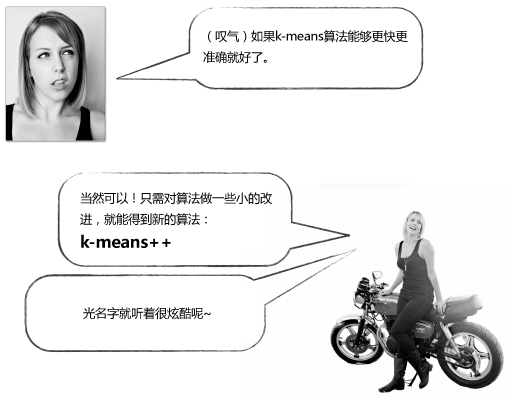### k-means++

1. 随机选取一个点；
2. 重复以下步骤，直到选完k个点：
1. 计算每个数据点（dp）到各个中心点的距离（D），选取最小的值，记为D(dp)；
2. 根据D(dp)的概率来随机选取一个点作为中心点。Dc1表示到中心点1的距离，Dc2表示到中心点2的距离。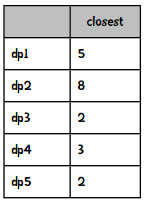``````data = [('dp1', 0.25), ('dp2', 0.4), ('dp3', 0.1),
('dp4', 0.15), ('dp5', 0.1)]
``````

``````import random
random.seed()

def roulette(datalist):
i = 0
soFar = datalist
ball = random.random()
while soFar < ball:
i += 1
soFar += datalist[i]
return datalist[i]
``````

``````import collections
results = collections.defaultdict(int)
for i in range(100):
results[roulette(data)] += 1
print results

{'dp5': 11, 'dp4': 15, 'dp3': 10, 'dp2': 38, 'dp1': 26}
``````

k-means++选取起始点的方法总结下来就是：第一个点还是随机的，但后续的点就会尽量选择离现有中心点更远的点。``````self.centroids = [[self.data[i][r] for i in range(1, len(self.data))]
for r in random.sample(range(len(self.data)),
self.k)]
``````

``````self.selectInitialCentroids()
````````````def distanceToClosestCentroid(self, point, centroidList):
result = self.eDistance(point, centroidList)
for centroid in centroidList[1:]:
distance = self.eDistance(point, centroid)
if distance < result:
result = distance
return result

def selectInitialCentroids(self):
"""实现k-means++算法中的起始点选取过程"""
centroids = []
total = 0
# 首先随机选取一个点
current = random.choice(range(len(self.data)))
centroids.append(current)
# 开始选取剩余的点
for i in range(0, self.k - 1):
# 计算每个点到最近的中心点的距离
weights = [self.distanceToClosestCentroid(x, centroids)
for x in range(len(self.data))]
total = sum(weights)
# 转换为权重
weights = [x / total for x in weights]
# 开始随机选取
num = random.random()
total = 0
x = -1
# 模拟轮盘游戏
while total < num:
x += 1
total += weights[x]
entroids.append(x)
self.centroids = [[self.data[i][r]  for i in range(1, len(self.data))]
for r in centroids]
``````